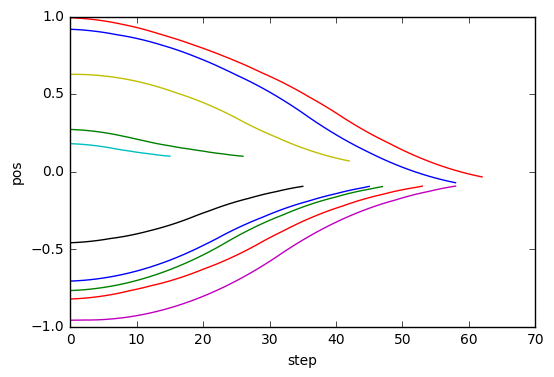# Introduction

For those who want to try reinforcement learning, but want to implement the algorithm by themselves ... We will prepare an environment for the original subject and explain the flow of reinforcement learning with keras-rl.

# Runtime environment

• Python 3.5
• keras 1.2.0
• keras-rl 0.2.0rc1
• Jupyter notebook

# Library to use

keras

https://github.com/fchollet/keras

``````pip install keras
``````

It is a deep learning framework that is talked about when it is easy to build a network.

keras-rl https://github.com/matthiasplappert/keras-rl

It is a library that implements deep reinforcement learning algorithms such as DQN using keras. See here for supported algorithms. Clone and install the git repository.

``````git clone https://github.com/matthiasplappert/keras-rl.git
pip install ./keras-rl
``````

OpenAI gym https://github.com/openai/gym

``````pip install gym
``````

A library with various environments for reinforcement learning. Keras-rl requires a gym interface for the reinforcement learning environment, so install it. There is a code to learn gym CartPole with DQN in keras-rl example, so give it a try Let's do it.

# Building an environment for reinforcement learning

The reinforcement learning environment that keras-rl learns implements Env of OpenAI gym. In the comment of Env of gym to implement, (https://github.com/openai/gym/blob/master/gym/core.py#L27)

``````    When implementing an environment, override the following methods
_step
_reset
_render
_close
_configure
_seed
And set the following attributes:
action_space: The Space object corresponding to valid actions
observation_space: The Space object corresponding to valid observations
reward_range: A tuple corresponding to the min and max possible rewards
``````

Although it is written, at a minimum, it is OK if the following is implemented.

``````_step
_reset
action_space
observation_space
``````

This time, we will take a simple example of a point that moves on a straight line, and let's take an example of manipulating the velocity from a random initial position and aiming to reach the origin.

``````import gym
import gym.spaces
import numpy as np

#Manipulate the speed of points moving on a straight line to reach a goal(origin)Environment whose goal is to move to
class PointOnLine(gym.core.Env):
def __init__(self):
self.action_space = gym.spaces.Discrete(3) #Action space. 3 types of speed reduction, as it is, increase

high = np.array([1.0, 1.0]) #Observation space(state)Dimension(Two dimensions of position and velocity)And their maximum
self.observation_space = gym.spaces.Box(low=-high, high=high) #The minimum value is minus the maximum value

#Called for each step
#Implemented to take action and return the next state, reward, and episode
def _step(self, action):
#Receive action and determine next state
dt = 0.1
acc = (action - 1) * 0.1
self._vel += acc * dt
self._vel = max(-1.0,  min(self._vel, 1.0))
self._pos += self._vel * dt
self._pos = max(-1.0,  min(self._pos, 1.0))

#Episode ends when the absolute values of position and velocity are small enough
done = abs(self._pos) < 0.1 and abs(self._vel) < 0.1

if done:
#Positive reward when finished
reward = 1.0
else:
#Negative reward over time
#If you reduce the absolute value as the distance gets closer so that you get closer to the goal, learning will proceed faster.
reward = -0.01 * abs(self._pos)

#Returns next state, reward, whether finished, additional information
#Empty dict as there is no additional information
return np.array([self._pos, self._vel]), reward, done, {}

#Called at the beginning of each episode and implemented to return the initial state
def _reset(self):
#Initial state is random position, zero velocity
self._pos = np.random.rand()*2 - 1
self._vel = 0.0
return np.array([self._pos, self._vel])
``````

# Building and learning DQN

Refer to dqn_cartpole.py of keras-rl example for the code to build and learn DQN. write.

``````from keras.models import Sequential
from keras.layers import Dense, Activation, Flatten
from keras.optimizers import Adam

from rl.agents.dqn import DQNAgent
from rl.policy import EpsGreedyQPolicy
from rl.memory import SequentialMemory

env = PointOnLine()
nb_actions = env.action_space.n

#DQN network definition
model = Sequential()
print(model.summary())

#memory for experience replay
memory = SequentialMemory(limit=50000, window_length=1)
#The action policy is orthodox epsilon-greedy. In addition, Boltzmann QPolicy, which determines the probability by the Q value of each action, is available.
policy = EpsGreedyQPolicy(eps=0.1)
dqn = DQNAgent(model=model, nb_actions=nb_actions, memory=memory, nb_steps_warmup=100,
target_model_update=1e-2, policy=policy)

history = dqn.fit(env, nb_steps=50000, visualize=False, verbose=2, nb_max_episode_steps=300)
#If you want to draw the state of learning, in Env_render()Implement and visualize=True,
``````

# Drawing tests and results

Test the learned Agent and try drawing the results. Implement Callback to store information for each step (not in keras-rl?), Execute test and plot the result accumulated in Callback.

``````import rl.callbacks
class EpisodeLogger(rl.callbacks.Callback):
def __init__(self):
self.observations = {}
self.rewards = {}
self.actions = {}

def on_episode_begin(self, episode, logs):
self.observations[episode] = []
self.rewards[episode] = []
self.actions[episode] = []

def on_step_end(self, step, logs):
episode = logs['episode']
self.observations[episode].append(logs['observation'])
self.rewards[episode].append(logs['reward'])
self.actions[episode].append(logs['action'])

cb_ep = EpisodeLogger()
dqn.test(env, nb_episodes=10, visualize=False, callbacks=[cb_ep])

%matplotlib inline
import matplotlib.pyplot as plt

for obs in cb_ep.observations.values():
plt.plot([o for o in obs])
plt.xlabel("step")
plt.ylabel("pos")
``````
``````Testing for 10 episodes ...
Episode 1: reward: 0.972, steps: 17
Episode 2: reward: 0.975, steps: 16
Episode 3: reward: 0.832, steps: 44
Episode 4: reward: 0.973, steps: 17
Episode 5: reward: 0.799, steps: 51
Episode 6: reward: 1.000, steps: 1
Episode 7: reward: 0.704, steps: 56
Episode 8: reward: 0.846, steps: 45
Episode 9: reward: 0.667, steps: 63
Episode 10: reward: 0.944, steps: 29
``````I was able to learn to go smoothly to position 0.